﻿ 高压静电场对循环冷却水阻垢研究 Scale Inhibition Effects of High Voltage Electrostatic Fields in Circulating Cooling Water System

Applied Physics
Vol. 09  No. 03 ( 2019 ), Article ID: 29113 , 6 pages
10.12677/APP.2019.93014

Scale Inhibition Effects of High Voltage Electrostatic Fields in Circulating Cooling Water System

Yun Jia, Zhihao Zhang, Judong Zhao*, Yuquan Wang, Lei Gao

Department of Physics, College of Science, Inner Mongolia University Technology, Hohhot Inner Mongolia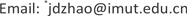Received: Feb. 13th, 2019; accepted: Feb. 25th, 2019; published: Mar. 4th, 2019ABSTRACT

The scale inhibition effect of self-made water was studied by using dynamic simulation bench of industrial circulating cooling water and high voltage electrostatic water processor. The results showed that the scale inhibition rate calculated by the gravimetric method was 50.0% after 10 hours of operation at a working voltage of 4.0 kV. The smaller the concentration of Ga2+ in the circulating water, the larger the amount of fouling, and the experiment showed that the amount of fouling was the highest two hours before the action.

Keywords:High Voltage Electrostatic Field, Circulating Cooling Water, Scale Inhibition RateCopyright © 2019 by author(s) and Hans Publishers Inc.1. 引言

2. 实验部分

2.1. 实验装置及方法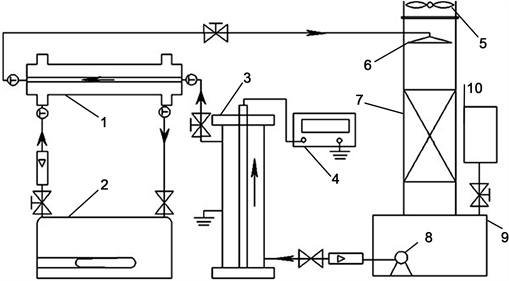1-换热器；2-恒温水浴锅；3-高压静电水处理器；4-高压电源；5-轴流风机；6-喷头；7-冷却塔；8-循环水泵；9-集水池；10-补水系统。

Figure 1. Flow chart of experiment of scale inhibition 

2.2. 实验水质

2.3. CaCO3的结晶过程

$\begin{array}{l}{\text{CaCl}}_{\text{2}}\to {\text{Ca}}^{\text{2+}}{\text{+2Cl}}^{-}\\ {\text{NaHCO}}_{\text{3}}\to {\text{Na}}^{\text{+}}{\text{+HCO}}_{\text{3}}^{-}\\ {\text{HCO}}_{\text{3}}^{-}\to {\text{H}}^{\text{+}}{\text{+CO}}_{\text{3}}^{2-}\\ {\text{Ca}}^{\text{2+}}{\text{+CO}}_{\text{3}}^{2-}{\text{=CaCO}}_{3}^{-}\end{array}$

CaCO3溶解度很小，属难溶性盐，当液相成垢离子的浓度达到饱和状态后在换热器表面上形成CaCO3水垢。

3. 实验结果与分析

 (1)

$k=\frac{{m}_{2}-{m}_{1}}{{m}_{2}}$ (2)

${m}^{\text{'}}{}_{2}$ ——未处理组的附着垢量，g；

${m}_{1}$ ——处理组的总垢量，g；

${m}_{2}$ ——未处理组的总垢量，g。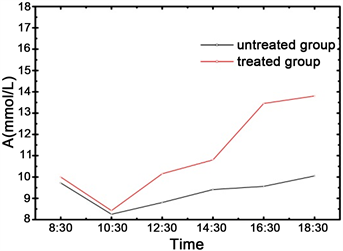Figure 2. Calcium hardness [Ca2+] history curve of treated and untreated group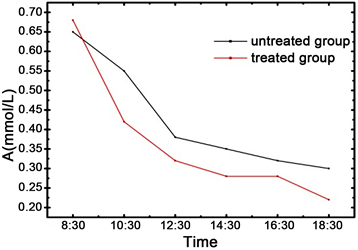Figure 3. Alkalinity [ ${\text{HCO}}_{\text{3}}^{-}$ ] history curve of treated untreated group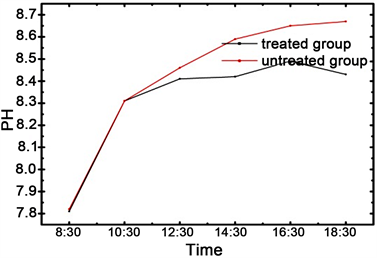Figure 4. PH value history curve of treated and untreated group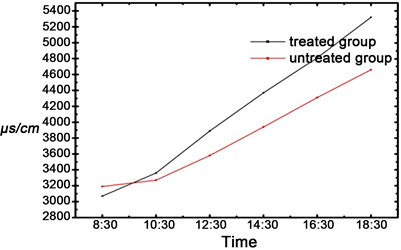Figure 5. Conductivity history curve of treated and untreated group

4. 结论

Scale Inhibition Effects of High Voltage Electrostatic Fields in Circulating Cooling Water System[J]. 应用物理, 2019, 09(03): 121-126. https://doi.org/10.12677/APP.2019.93014

1. 1. 能源部西安热工研究所. 热工技术手册(电厂化学) [M]. 北京: 水利电力出版社, 1993: 16-18.

2. 2. 杨星, 刘智安, 龙山, 等. 电磁场协同处理电厂循环冷却水阻垢实验[J]. 工业水处理, 2016, 36(5): 44-66.

3. 3. 牛慧杰, 刘智安, 赵巨东, 等. 脉冲磁场阻垢处理器腔内磁感应强度的分布与计算[J]. 内蒙古师范大学学报(自然科学汉文版), 2015, 6(44): 782-784.

4. 4. 刘智安. 电厂水处理技术[M]. 北京: 中国水利水电出版社, 2009: 6-15.

5. 5. 王兴国. 火电厂水处理与化学监督[M]. 北京: 化学工业出版社, 2008: 26-32.

6. 6. 惠希增. 变频电磁场处理油田水防垢技术研究[D]: [硕士学位论文]. 北京: 中国石油大学, 2007.

7. 7. 王建国, 何芳, 邸昊, 等. 电磁场对结垢过程中电导率及PH值的影响[J]. 化学工程, 2013, 41(1): 32-36.

8. 8. 相亚军, 纪利春. 磁电协同用于电厂循环冷却水阻垢实验[J]. 化学工程, 2014, 42(10): 11-14.

9. 9. 姜德宁, Sintayehu, Z.D., 曹继华. 交变磁场对换热器阻垢效果的研究[J]. 中国给水排水, 2008, 24(7): 57-59.

10. 10. 张旭, 刘智安, 赵巨东, 等. 磁电复合式循环冷却水阻垢处理装置及阻垢实验研究[J]. 工业水处理, 2013, 33(10): 34-36.

11. 11. 中华人民共和国国家发展和改革委员会. 冷却水动态模拟实验方法(HG/T2160-2008) [S]. 北京: 化学工业出版社, 2008: 2-4.

12. 12. 安慧凤, 刘智安, 赵巨东, 等. 高压静电场对火电厂循环冷却水阻垢效果及机理[J]. 环境工程学报, 2013, 7(11): 4295-4299.

13. NOTES

*通讯作者。